# Time Conversion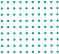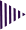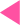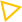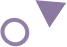=
Best Time conversion calculator

# Online time conversion calculator

This time converter Calculator lets you either enter the time value and units you wants to convert from, or choose the time unit you wish to convert to. You can converts seconds, Millisecond’s, microseconds, nanoseconds ,days, hours, weeks, months, and years. Months are calculated in this time convert by taking the average of the last 12 months.

### How do you calculate Time conversion?

The term unit of conversion refers to a process involving multiplication or division by a numerical factors. Weights, distances, and temperatures can all be measured in a variety of ways. In math’s or other subjects, or day-to-day living, the Knowledge of units of conversion is useful.

### Units of Time Conversion Chart

1. 1 hour = 60 minutes.
2. 1 minute = 60 seconds.
3. 1 hour = 60 minutes = 3600 seconds (60 × 60)
4. 1 day = 24 hours.
5. 1 week = 7 days.
6. 1 year = 365 days.
7. 1 year = 12 months.
8. 1 year = 52 weeks.

• Seconds Converts definition:-

In the international system of units, the second is the base unit of time. Convert any time Value to Seconds Using Multiplication. Example:- Convert 120 minutes into Seconds Multiply 120 minutes by 60 seconds per minute 120 min*60s/min=7200 seconds example: convert 28,800 seconds into hours Multiply 28,800 seconds by 12 hours per day 12 hours*12 hours per day = 3,732 hours

• Minute converter definition:-

-A period of time equal to sixty seconds or 1/60th of an hour. 120 minutes equals 2 hours because 120/60=2. You can convert minutes to hours by dividing the number of minutes by 60.

• Hour converter definition:-

- An hour is a period of sixty minutes. Divided into 60 minutes, it is the twenty-fourth part of a day and night. As there are 60 minutes in an hours, that’s the ratio used in the formula to convert 90 minutes to hours. 90 min = (90 ÷ 60) = 1.5 hr.

• Day converter definition:-

-A day is a unit of time that is equivalent to 24 hours period of time or the period of light between sunrise and sunset. There are 365 days in a year. Days are equal to the hours divided by 24. To convert days from hours to days, use the formula below: 120hr = (120 ÷ 24) = 5 days.

• Year converter definition:-

-A year is a unit of time that is equal to twelve months or 365 or 366 days or 52 weeks, which begins on the first of January and ends on the 31st of December
What is the use of year?
Age is measured by the year. In the case of someone or something that has been alive or existing for twenty years, they have lived or existed for twenty years. An example of a year is January 1, 2022 through December 31, 2022.
How do you calculate month to year
The time in years is equal to the months divided by 12. As an example, here is how you could convert 24 months to year by using the formula above. 24 month = (24 ÷ 12) = 2 Year